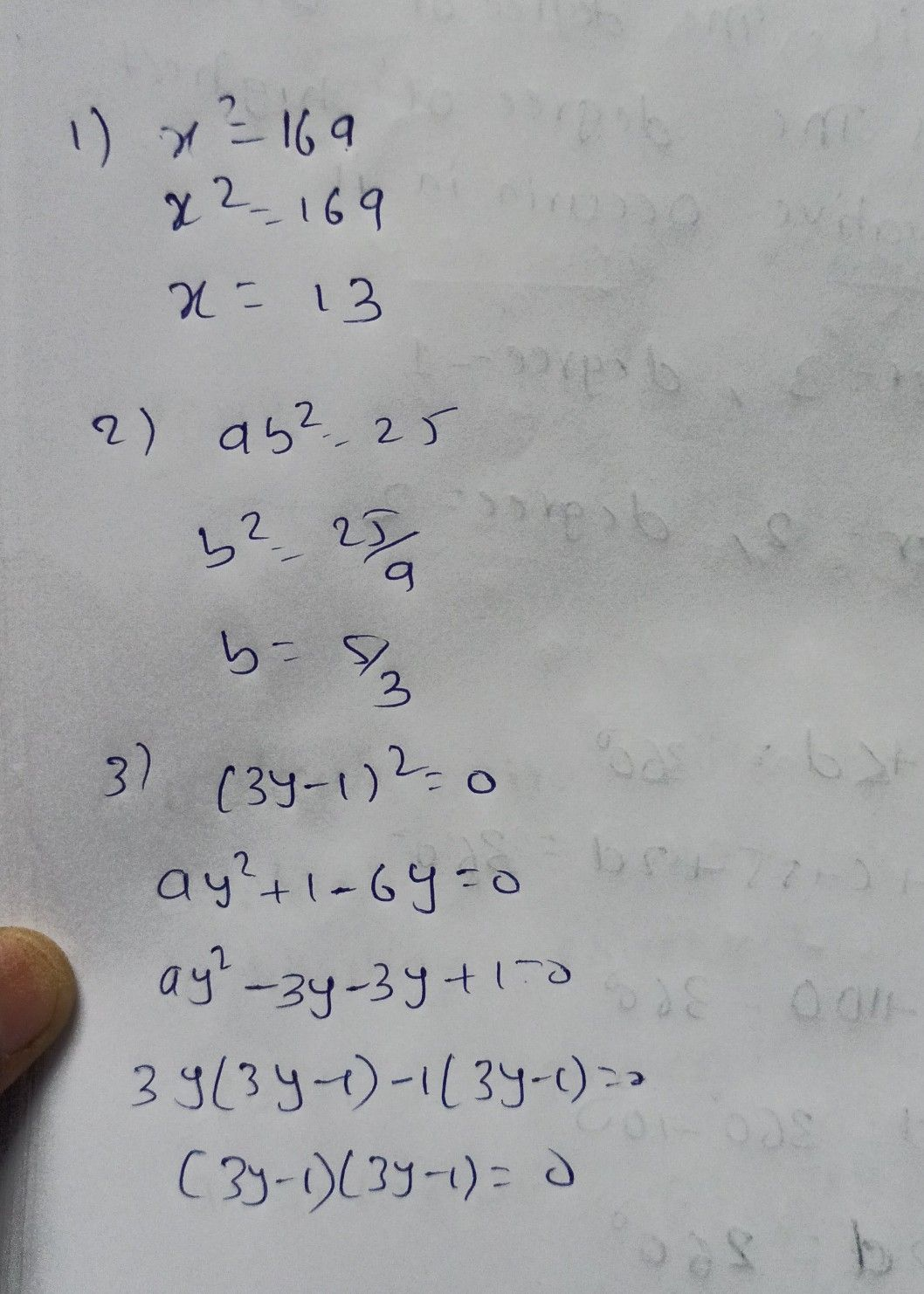Symbol
ProblemLearning Task $3.$ Solve for the variable of the following quadratic equations A. by extracting square roots. $1$ $x^{2}=169$ $4$ $\left(x-2\right)^{2}=16$ $2$ $9b2=25$ $5$ $2\left(t-3\right)^{2-72}=0$ $3$ $\left(3y-1\right)^{2}=0$
7th-9th grade
Algebra
Search count: 112
Question content
please help me
SolutionQanda teacher - tejasriStudent
thank you so muchQanda teacher - tejasri
plz give rating
Dear, If you have any doubt feel free to ask:) Please evaluate and give GIFT coins: 1. Click on top right corner EVALUATE button. 2. Select 5 star. 3. Click on gift coins + three times(C3000)(NOT REDUCE YOUR COINS). 4. Click on DONE button on top right corner.
please